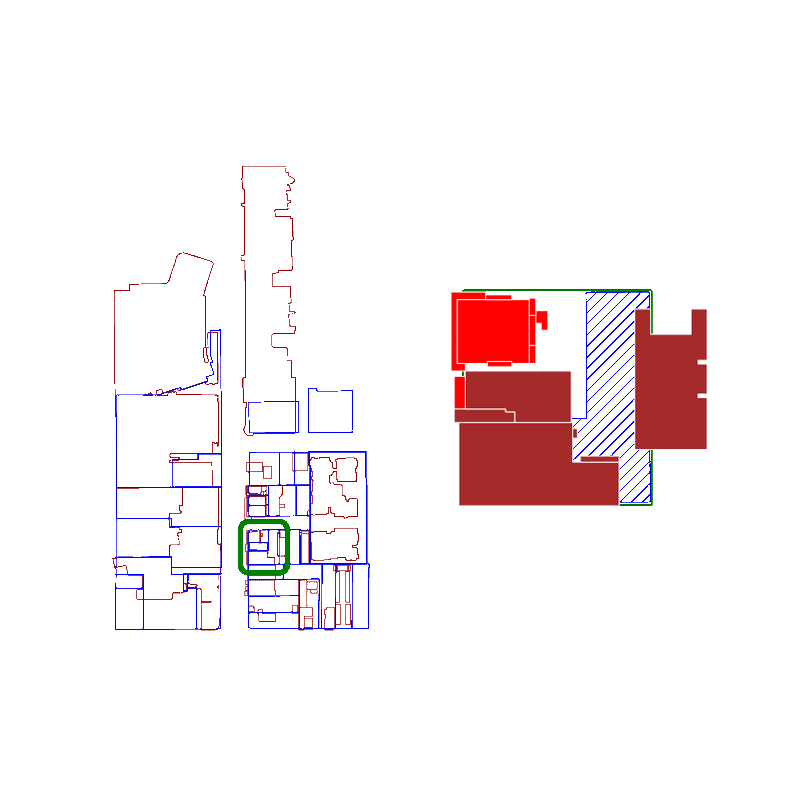# Using the GeoPandas Spatial Index to Fix Slow Intersections## Brendan Ashworth

September 6, 2022

When merging two spatial datasets together, using the GeoPandas spatial index is a great way to accelerate the calculation of intersections. This allows for easy joins, for example: for every real estate parcel, give me a list of buildings that intersect with it.

Naively calculating the intersection of all buildings with each parcel creates an `O(n^2)` algorithm that tends to blow up as your datasets get larger. This typically looks like this:

``````for i, specific_parcel in tqdm(parcels.iterrows(), total=len(parcels)):
# run intersection for every building<->parcel pair, O(n^2)
is_intersection = buildings.geometry.intersects(specific_parcel.geometry)
overlapping_buildings = buildings.loc[is_intersection]
100%|███████████████████████████████████████| 3497/3497 [00:37<00:00, 92.34it/s]``````

If we index data as we load it, we scale with `O(n log n)` because a lookup in an R-tree is much faster than looping through all elements.

The default spatial index operates on bounding boxes. Here's an example of using the spatial index to calculate intersections much more quickly:

``````# build spatial index
spatial_index = buildings.sindex

for i, specific_parcel in tqdm(parcels.iterrows(), total=len(parcels)):
# spatial_index.query() for every parcel is O(n log n)
possible_matches_index = list(spatial_index.query(specific_parcel.geometry))
possible_matches = buildings.iloc[possible_matches_index]
# run true intersection query only on possible matches
actual_matches = possible_matches[possible_matches.intersects(specific_parcel.geometry)]
100%|██████████████████████████████████████| 3497/3497 [00:10<00:00, 328.92it/s]``````

In this case, using the spatial index was 3x faster than the naive loop. These benefits are of course more important the larger a dataset you have, and spatial datasets quickly grow into millions of rows.

When you query a spatial index for possible matches, you're actually searching for an approximate list of polygons that intersect with the original polygon's bounding box.

Because we're using bounding boxes to approximate intersections at first, the spatial index behaves best when the polygons are approximately square. The worst performance is if the bounding box is significantly larger than the area of the polygon.

Here is an animation where we show false positives in red and true positives in brown:For real estate applications, the GeoPandas spatial index provides a faster way to decompose adjacent geometries into relevant groups.

If you're interested in learning more, Geoff Boeing has a great post where he splits up polygons into smaller squares, which have bounding boxes that more closely follow the initial shape. This accelerates using a spatial index because it decreases the false positive rate.